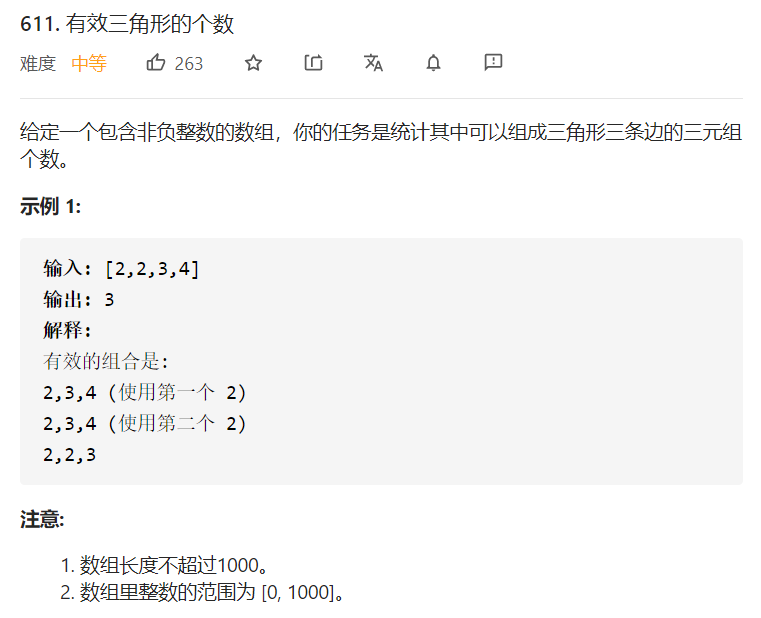5G时代下一个聚合的编程学习网

# 力扣611 有效三角形的个数### 排序 + 双指针

``````class Solution {
public:
int triangleNumber(vector<int>& nums) {
sort(nums.begin(),nums.end());
int res = 0;
for(int i = 0; i < nums.size(); ++i) {
// 遍历第一条边
int j = i+1;              // 第二条边的下标
int k = j;                // 第三条边没找到时的下标
for(;j < nums.size(); ++j){
// 遍历第二条边
k = max(k,j);         // 上次没找到第三条边时，将第三条边初始化为当前第二条边
while(k + 1 < nums.size()){
if(nums[i] + nums[j] > nums[k + 1])  ++k;
else break;
}
res += k - j;         // (j,k] 共有 k - j 个元素
}
}
return res;
}
};
``````